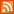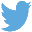Bucaro TecHelpHTTPS Encryption not required because no account numbers orpersonal information is ever requested or accepted by this siteCustom Search# The for Loop

A loop structure allows you execute a series of statements over and over. Several kinds of loop structures are available in Java Script, the most common loop is the for loop, which enables you to execute the loop a specific number of times.

The control statement of the loop has three sections, the initialization expression, the comparison test and the update expression as shown below.

```for(initialization; comparison; update)
{
statements;
}
```

The initialization expression is where you perform any initialization required for the loop, usually you create and initialize the loops counter or index variable here.

The update expression is the third section of the for loop's statement. Here, you usually increment the loops counter or index, which tracks how many times the statements in the body of the loop have been executed.

The comparison test is where you evaluate or test the loops counter or index to determine if the statements in the body of the loop have been executed the required number of times, called iterations.

```for (var i = 1; i <= 10; i++)
{
document.write("This is iteration number " + i + "<br />");
}
```

The for loop shown above initializes the variable i to 1, increments i by 1 on each iteration of the loop, and performs the comparison test i <= 10 is i less-than or equal-to 10 to determine if the loop has executed 10 times. The statement in the body of the loop prints the value of the loop's control variable on each iteration as shown below.

```This is iteration number 1
This is iteration number 2
This is iteration number 3
This is iteration number 4
This is iteration number 5
This is iteration number 6
This is iteration number 7
This is iteration number 8
This is iteration number 9
This is iteration number 10
```

The loop's control variable can be initialized to any number, incremented by any value and tested for any condition. The loop shown below initializes the variable j to 4, increments j by 2 on each iteration, and performs the test j <= 12 to determine if the loop has executed the required number of times.

```for (var j = 4; j <= 12; j = j + 2)
{
document.write("This is iteration number" + j + "<br />");
}
```

The statement in the body of the loop prints the value of the loop's control variable as shown below.

```This is iteration number 4
This is iteration number 6
This is iteration number 8
This is iteration number 10
This is iteration number 12
```

The loop shown below initializes the variable k to 10, decrements k by 1 on each iteration, and performs the test j > 0 to determine if the loop has executed the required number of times.

```for (var k = 10; k > 0; k--)
{
document.write("The value of k is " + k + "<br />");
}
```

The statement in the body of the loop prints the value of the loop's control variable as shown below.

```The value of k is 10
The value of k is 9
The value of k is 8
The value of k is 7
The value of k is 6
The value of k is 5
The value of k is 4
The value of k is 3
The value of k is 2
The value of k is 1
```

 .menubtn { margin-top:10px; margin-left:50px; width:220px; opacity:1; border-style:solid; border-color:#009000; background-color:#66ff66; transition-property: opacity; transition-duration: 1s; transition-timing-function: ease-out; } .menubtn:hover { opacity:1; background-color:#e5ff23; } Menu - More Java Script Quick ReferenceRSS FeedFollow @Stephen Bucaro[Site User Agreement] [Privacy Policy] [Site map] [Search This Site] [Contact Form]
Copyright©2001-2023 Bucaro TecHelp 13771 N Fountain Hills Blvd Suite 114-248 Fountain Hills, AZ 85268